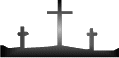```BIBLETRUTHONLINE.COM```
```

```
```  Understanding the Theory of Expounded Numbers
```
```
We will talk frequently in terms of numbers and their meaning in the
bible.  We have formed a theory of expounded numbers (expounded means:
to explain or clarify).  This theory is based on the simplest form of
addition that we will now explain in detail.

Think of numbers as a unit in this exercise.

A pyramid structure will be used for simplicity:

X           1 (one unit)

X X          2 (two units)

X X X         3 (three units)

X X X X        4 (four units)

X X X X X       5 (five units)

X X X X X X      6 (six units)

X X X X X X X     7 (seven units)

X X X X X X X X    8 (eight units)

X X X X X X X X X   9 (nine units)

X X X X X X X X X X  10(ten units)

O.K. Lets look at the first three rows of the pyramid:

X   1 (one unit = one unit)

X X  2 (two units = a total of three units)

X X X 3 (three units = a total of six units)

How is it that three units equals six units? Count how many X's there are. . .

So, at number 8 how many total units are there?

X

X X

X X X

X X X X

X X X X X

X X X X X X

X X X X X X X

X X X X X X X X

THERE ARE A TOTAL OF 36 X'S IN THE ABOVE EXAMPLE.

8 = 36

Another easy way of adding this way is to take a calculator and add

1 + 2 + 3 + 4 + 5 . . . and use the numbers that appear as answers.

Here is a table for numbers 1 through 9 (the simple numbers).

ONE     1 +
TWO     2 = 3
THREE   3 = 6
FOUR    4 = 10
FIVE    5 = 15
SIX     6 = 21
SEVEN   7 = 28
EIGHT   8 = 36
NINE    9 = 45

So, when we say 9 expounded = 45, it will be clear as to what we
mean by it.

Back to Truth for Future Lessons >>
```